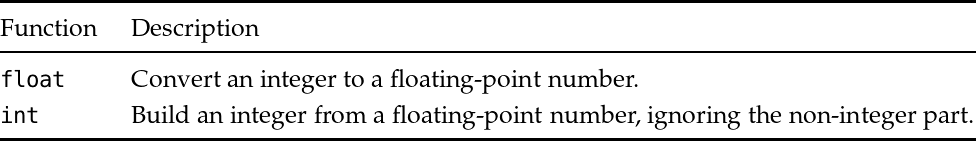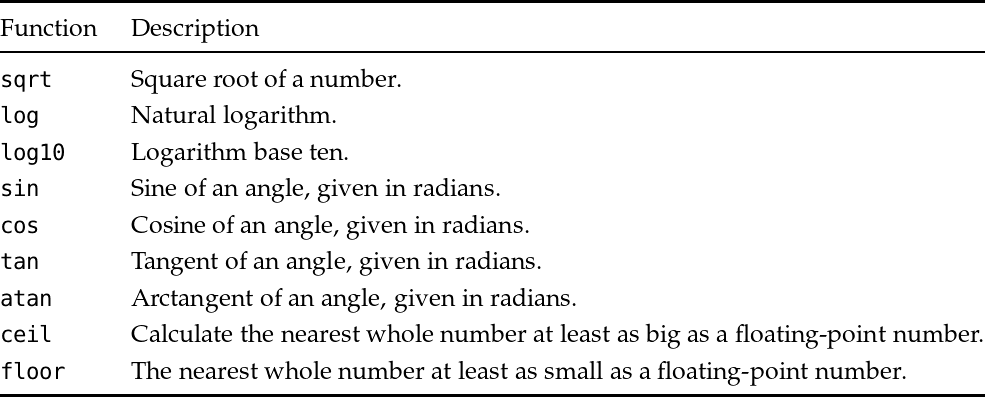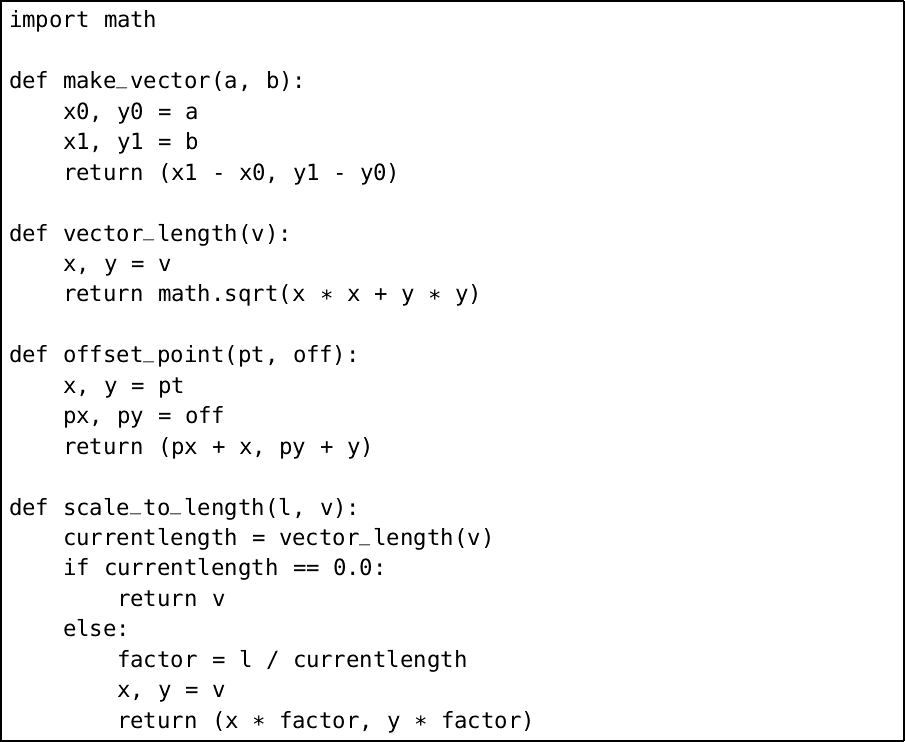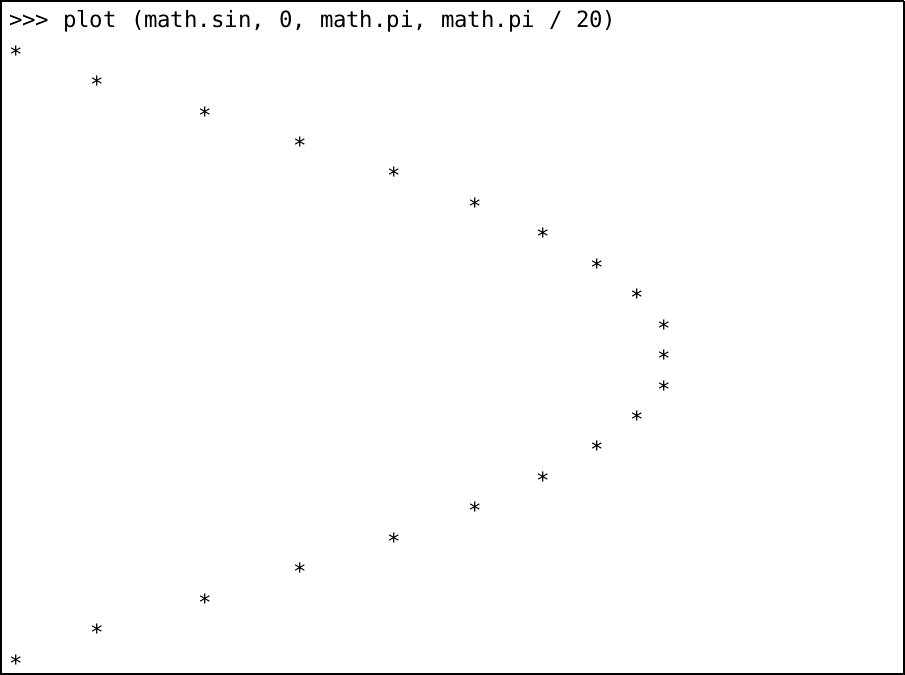Back to Contents

# 10. The Other Numbers

The only numbers we have considered until now have been the whole numbers, or integers. For a lot of programming tasks, they are sufficient. And, except for their limited range and the possibility of division by zero, they are easy to understand and use. However, we must now consider the real numbers.

### Introducing floating-point numbers

It is clearly not possible to represent all numbers exactly – they might be irrational like π or e and have no finite numerical representation. For most uses, a representation called floating-point is suitable, and this is how Python’s real numbers are stored. Not all numbers can be represented exactly, but arithmetic operations are very quick.

We can write a floating-point number by including a decimal point somewhere in it. For example `1.6` or `2.`  or `386.54123`. Negative floating-point numbers are preceded by the `-` character just like negative integers. Here are some floating-point numbers in Python:

``````Python
>>> type(1.5)
<class 'float'>
>>> 6.
6.0
>>> -2.3456
-2.3456
>>> 1.0 + 2.5 * 3.0
8.5
>>> 1.0 / 1000.0
0.001``````

### Mixing different kinds of number

When we mix integers and floating-point numbers, Python will automatically convert the integer to a floating point so that the operation can work:

``````Python
>>> 1 + 2 * 3.0
7.0``````

Here the integer `2` is converted to the floating-point number `2.0` for the multiplication, which results in the floating-point result `6.0`. Then the integer `1` must be similarly converted to a floating-point number to do the addition and produce the final result. The conversion only happens when the expression requires it:

``````Python
>>> type(1 + 2)
<class 'int'>
>>> type(1 + 2.0)
<class 'float'>``````

Sometimes an operation on two integers can produce a floating-point result, for example using the division operator:

``````Python
>>> 1 / 2
0.5``````

You can see now why we introduced addition, subtraction, and multiplication in chapter 1, but left out division. There is an integer division operator too:

``````Python
>>> 2 // 3
0
>>> 10 // 5
2``````

You can see that this operator calculates just the whole part. We already have the `%` modulus operator to calculate the remainder.

### Limits of range and precision

Here is an example of the limits of precision in floating-point operations:

``````Python
>>> 3.123 - 3.0
0.12300000000000022``````

Very small or very large numbers are written using so-called scientific notation:

``````Python
>>> 1.0 / 100000.0
1e-05
>>> 30000. ** 10.
5.9049e+44``````

These are the numbers 1 × 10−5 and 5.9049 × 1044 respectively. We can find out the range of numbers available:

``````Python
>>> import sys
>>> sys.float_info.max
1.7976931348623157e+308
>>> sys.float_info.min
2.2250738585072014e-308``````

Working with floating-point numbers requires care, and a comprehensive discussion is outside the scope of this book. These challenges exist in any programming language using the floating-point system. We will leave these complications for now – just be aware that they are lurking and must be confronted when writing robust numerical programs.

### Standard functions

There are two built-in functions for converting between integers and floating-point numbers:Notice that `int` is not the expected rounding function:

``````Python
>>> float(2)
2.0
>>> int(2.3)
2
>>> int(2.8)
2``````

If we use `import`` math`, more functions are available:For example, we can calculate:

``````Python
>>> import math
>>> math.sqrt(3 * 3 + 4 * 4)
5.0
>>> math.sqrt(2)
1.4142135623730951``````

The ceiling and floor functions give us the rounding behaviour we expect:

``````Python
>>> math.ceil(2.3)
3
>>> math.floor(2.3)
2
>>> math.ceil(2.5)
3``````

Note that they return integers. But we can get back to floating-point easily, of course:

``````Python
>>> float(math.ceil(2.7))
3.0``````

### Example: vectors

Let us write some functions with floating-point numbers. We will write some simple operations on vectors in two dimensions. We will represent a point as a pair of floating-point numbers such as `(2.0, 3.0)`. We will represent a vector as a pair of floating-point numbers too. Now we can write a function to build a vector from one point to another, one to find the length of a vector, one to offset a point by a vector, and one to scale a vector to a given length:Notice that we have to be careful about division by zero, just as with integers. We have used tuples for the points because it is easier to read this way – we could have passed each floating-point number as a separate argument instead, of course.

Floating-point numbers are often essential, but must be used with caution. You will discover this when answering the questions for this chapter. Some of these questions require using the built-in functions listed in the table above.

## Common problems

We should never use floating-point numbers to represent currency. For example, selling 145 items at \$2.34:

``````Python
>>> 145 * 2.34
339.29999999999995``````

Instead, we can store the numbers as integer amounts of cents:

``````Python
>>> 145 * 234
33930``````

We only need consider dollars when formatting the number for printing, not when calculating with it.

Repeated calculations can lead to errors compounding. For example, repeated addition is not the same as multiplication when it comes to floating-point numbers:

``````Python
>>> x = 0.0
>>> for y in range(10):
...   x += 0.1
...
>>> x
0.9999999999999999
>>> 0.1 * 10
1.0``````

## Summary

We have filled in a gap in our knowledge of Python: how to use real numbers, or floating-point approximations of them. We have learned to be wary of them, and so to use them only when really needed. We looked at the wide range of standard functions for manipulating floating-point numbers, including the `floor` and `ceil` functions, and the `int` and `float` functions for converting between floating point numbers and integers.

In the next chapter we look at the Python Standard Library, Python’s collection of helpful modules, in more depth.

## Questions

1. Give a function which rounds a positive floating-point number to the nearest whole number, returning another floating-point number.

2. Write a function to find the point equidistant from two given points in two dimensions.

3. Write a function to separate a floating-point number into its whole and fractional parts. Return them as a tuple.

4. Write a function `star` which, given a floating-point number between zero and one, draws an asterisk to indicate the position. An argument of zero will result in an asterisk in column one, and an argument of one an asterisk in column fifty.

5. Now write a function `plot` which, given a function which takes and returns a real number, a start and end point, and a step size, uses `star` to draw a graph. For example we might see:Here, we have plotted the sine function on the range 0…π in steps of size π/20.